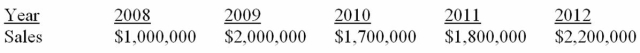# Finance Applications and Theory

## Quiz 15 :Financial Planning and ForecastingLooking for Finance Homework Help?

## Quiz 15 :Financial Planning and Forecasting

Showing 1 - 20 of 90Which of the following is a set of financial statements depicting an operating division of a firm's expected financial situation in the foreseeable future under the most reasonable set of assumptions concerning relevant factors?
Free
Multiple Choice

DThe set of assumptions underlying the firm's financial plan and the resulting projected financial statements are accordingly often referred to as which of the following?
Free
Multiple Choice

AFinancial planning involves estimating projected cash flows, which is useful for all the following except:
Free
Multiple Choice

DThe simplest approach to estimating a future period's sales is to assume that they will be equal to those of the latest observed period. In statistics, this is often simply referred to as which of the following?
Multiple ChoiceForecasted sales drives all of the following except:
Multiple ChoiceWhich of the following is defined as assuming that future sales will be equal to the average historical value across some relevant period?
Multiple ChoiceWhich of the following is used to remove the effects of seasonality from historic data?
Multiple ChoiceWhich of the following is the practice of one firm selling to another on credit terms?
Multiple ChoiceWhat is computed by dividing the amount of assets tied directly to sales (A*) by the amount of current sales (S0)?
Multiple ChoiceWhich of the following is the amount of external financing a firm must seek in order to change the asset base as necessary to support a different level of sales?
Multiple ChoiceWhich of the following can be computed as: Necessary increase in assets minus spontaneous increase in liabilities minus projected increase in retained earnings?
Multiple ChoiceIf a firm has excess capacity when calculating AFN (Additional Funds Needed), A* will most likely equal which of the following?
Multiple ChoiceWhich of the following are considered "chunky" or "lumpy" assets?
Multiple ChoiceThe additional funds needed by the firm can be calculated by assuming which of the following?
Multiple ChoiceWhich statement is most correct regarding how pro forma financial statements can be used to estimate additional funds needed?
Multiple ChoiceWhich of the following defines the term deseasonalize?
Multiple ChoiceWhich of the following defines MAPE?
Multiple ChoiceFirst order effects are defined as which of the following?
Multiple ChoiceSuppose a firm has had the historical sales figures shown below. What would be the forecast for next year's sales using the naïve approach?